# CAGR Formula Examples in Excel

CAGR in Excel is a formula that calculates the compound annual growth rate for any invested amount over the specified years or timeframe. Although there is no direct function in Excel that can help us identify the CAGR value, there are numerous functions available that can help us calculate the return rate on investment; for this, we need the number of payments to be made, as well as the current and future values of the invested money. If we compute CAGR using mathematical equations, the result will be the same as that determined using CAGR.

In this tutorial, you will thoroughly learn how to compute CAGR in MS Excel in seconds using many examples.

So, without further ado, let’s get started.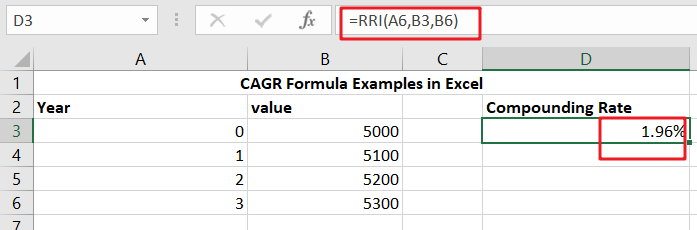## General Formula

To determine the yearly growth rate, divide the value of an investment at the end of the period by its value at the beginning of the period, multiply the result by an exponent of one divided by the number of years, and remove one.

`=(end/start)^(1/periods)-1`

## Summary

In Excel, there are various methods for calculating CAGR. The RRI function in Excel 2013 and later is the easiest approach. The formula in H9 in the case displayed is:

`=RRI(A6,B3,B6)`

## Explanation

As previously said, CAGR stands for Compound Annual Growth Rate. The CAGR is the average rate of return on investment over time. It is the rate of return necessary for an investment to increase from its starting balance to its ending ratio, provided earnings are reinvested annually and interest compounded annually.

Still confused? Don’t worry; by following the examples below, you will grasp how to utilize the CAGR formula in a step-by-step process:

### Excel CAGR Formula – Example #2

Let us create a ready-made formula in Excel that will compute the compound annual growth rate based on the variables we provide.

Step1: We require a starting value. So, in cell A1, type “Starting Value” as a heading.

Step2: There is an ending value. So, in Cell B1, enter “Ending Value” as the heading.

Step3: In cell C1, enter ” Number of Years ” as a heading for the total number of years.

Step4: In cell D1, enter a header for the final value and label it CAGR (Compound Annual Growth Rate).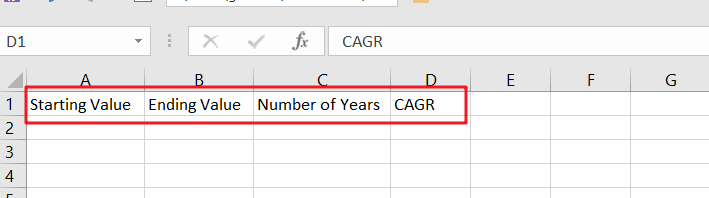Step5: In cell D2, enter the CAGR formula, which is,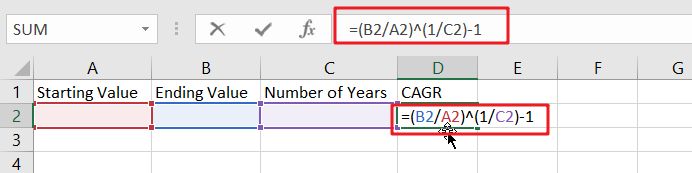Step6: Try placing any random value in the starting and ending values and the number of years. Cell D2 displays the CAGR.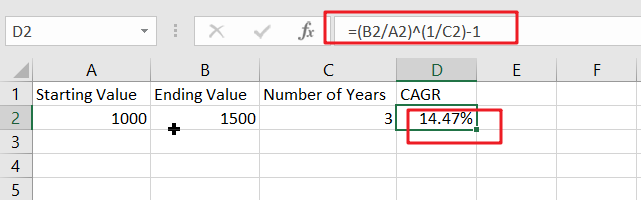### Excel CAGR Formula – Example #3

Let’s look at another CAGR Formula in the Excel sample. We have data below,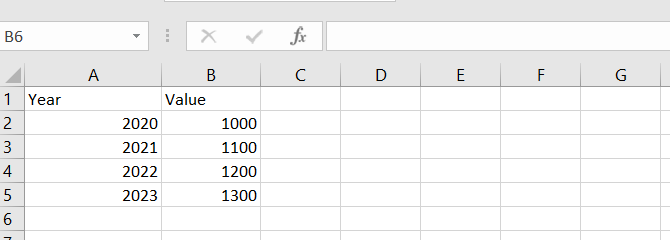Step1: Determine a starting value as the starting balance, which is B1.

Step2: As the Ending Balance, find an Ending Value, which is B5.

Step3: Determine the number of years, which is 3.

Step4: Enter the CAGR calculation in Cell C2 (Ending Balance/Starting Balance)(1/Number of Years) – 1.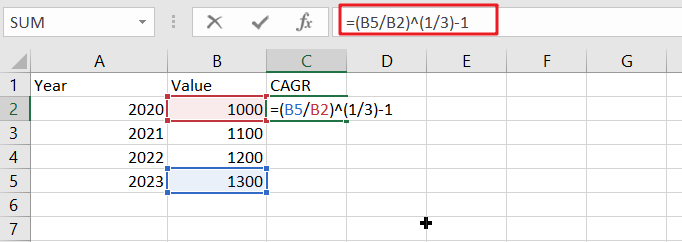Step5: The result will be as follows: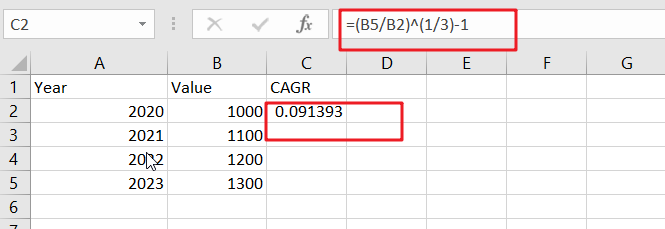Step6:To obtain the CAGR figure, click on the percent symbol in the Home Tab’s general area.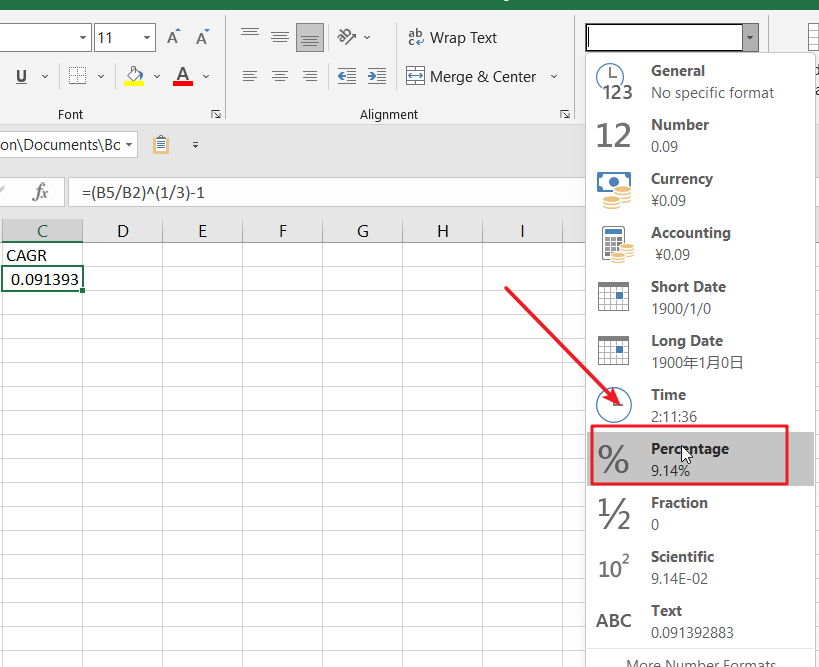Step7:The final result is 9.14%, representing the needed yearly growth rate.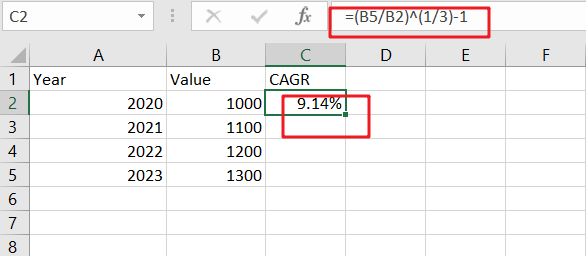In Excel, there are various methods for calculating CAGR:

## CAGR In Conjunction With The RRI Function

You may use the RRI function in Excel 2013 and later compute CAGR using a simple formula. H9 has the formula:

`=RRI(A6,B3,B6)`where B6 is the finishing value in year 3, B3 is the starting value, and A6 is the total number of periods.

Unlike most other financial functions, It is worth nothing in Excel; fv (future value, the third argument) does not have to be entered as a negative number in RRI.

## CAGR With A Manual Formula

CAGR may be calculated manually using the following formula:

`=(end/start)^(1/periods)-1`

The formula in E3 in the case illustrated is:

`=(B6/B3)^(1/A6)-1`

##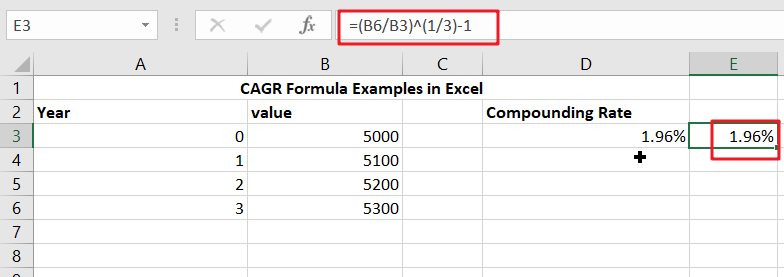B6 represents the finishing value in year 3, B3 represents the starting value or original investment, and A6 represents the total number of periods.

The first portion of the formula calculates total return, while the second part calculates annualized return throughout the life of the investment.

## Conclusion

The CAGR Formula in Excel computes the average yearly growth rate for a given time period. CAGR does not reflect the unevenness of growth rates in the intermediate years. It just smoothed the pace of the increase over time.

### Related Functions

• Excel RRI function
The Excel RRI function Returns an equivalent interest rate for the growth of an investment.The syntax of the RRI function is as below:The syntax of the RRI function is as below:= RRI (nper,pv,fv)